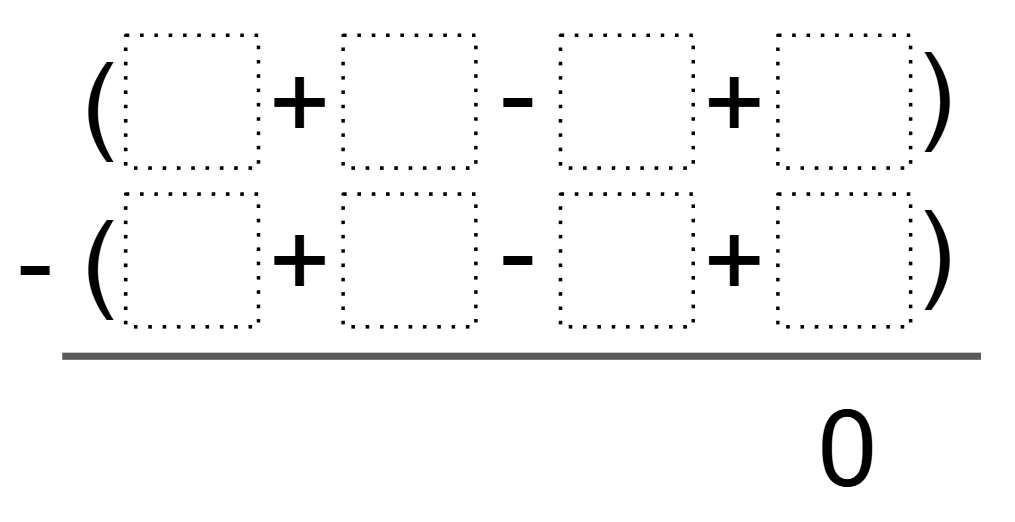Home > Grade 7 > Creating Zero

# Creating Zero

Directions: Using the digits 1 to 9 at most one time each, fill in the boxes to make the equality true:### Hint

What numbers will produce a difference of zero?

### Answer

Because of the commutative property, there are many different answer combinations. One possible solution would be: ( 9 + 6 – 5 + 2 ) – ( 8 + 7 – 4 + 1 )

Source: Bryan Anderson

## Converting a Fraction to a Decimal

Directions: Using the digits 1 to 9, at most one time each, place a digit …

### 3 comments

1.Also, (9+1-8+2) – (7+3-6+4) = 0.

•that would = -4

•you can use those numbers just move them around.